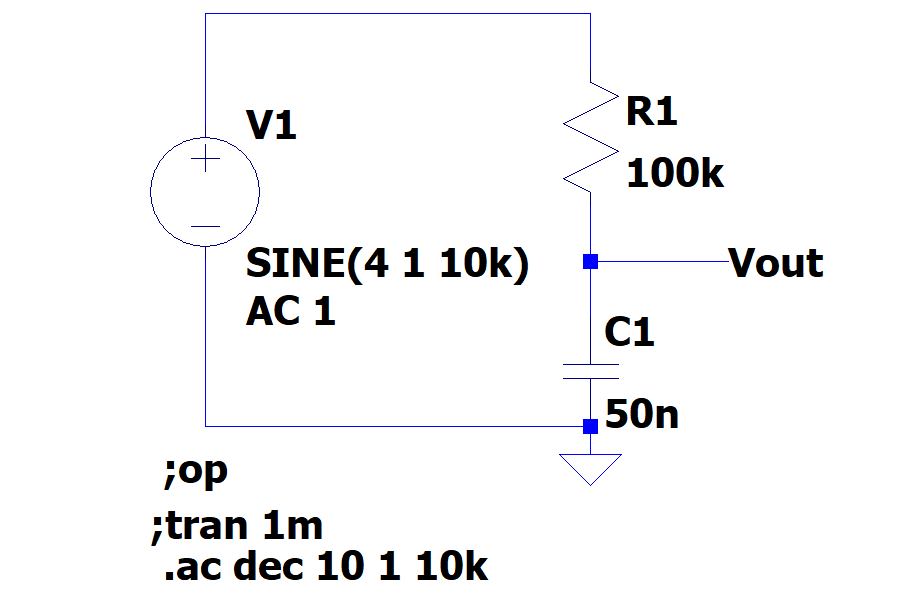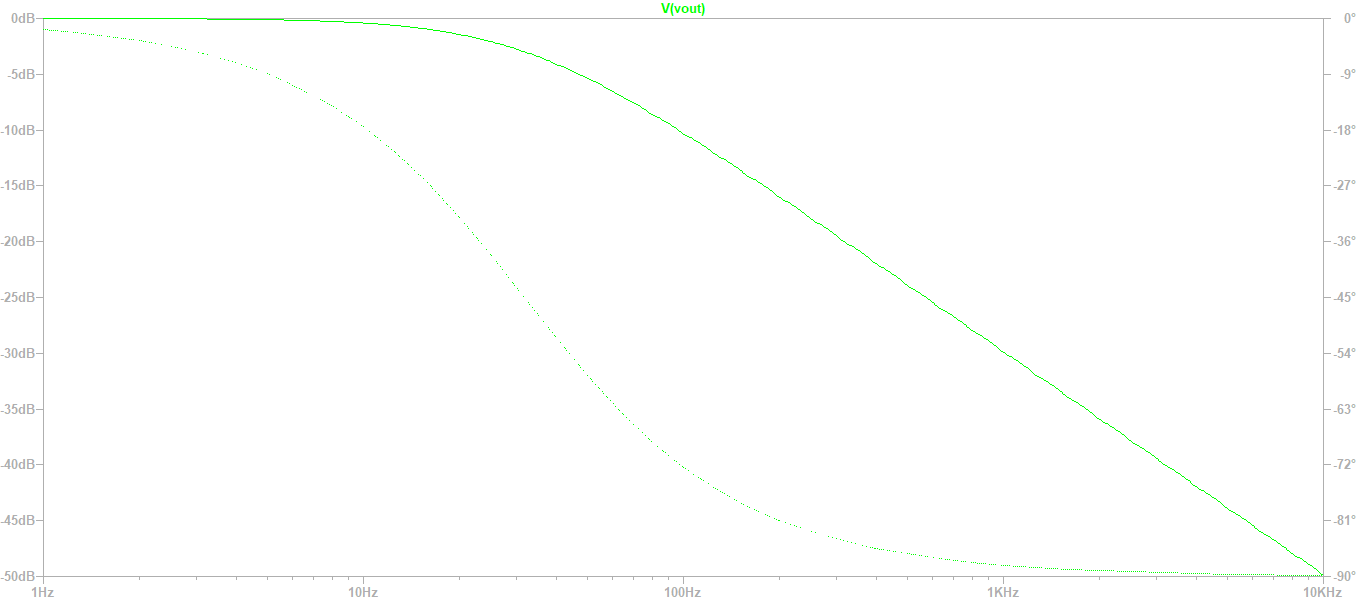# Overview

• Introduction
• Simulation directives

# Introduction

LTSPICE is a electronics simulation software that we will be using in this curse.
As introductory circuit, we drafted a basic RC circuit and simulated it in the frequency domain.
The plot shows the "Vout" output node.# Simulation directives

The software cannot do a simulation without instructions.
Located below the circuit there are directives which "say" what the simulation should do.
Here is an explanation of each directive used in the practice:
• op: calculates the DC voltage and current for each node fo the circuit.
• tran: defines the transient time simulation
• ac: calculates AC behavior depending on selectable paramaters